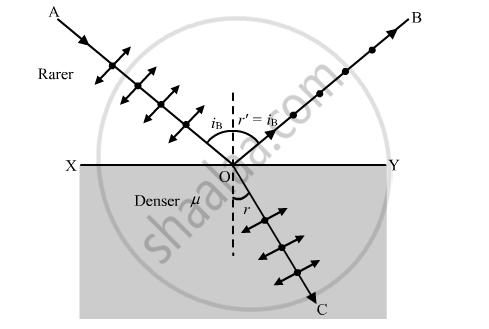# A Beam of Unpolarised Light is Incident on a Glass-air Interface. Show, Using a Suitable Ray Diagram, that Light Reflected from the Interface is Totally Polarised, - Physics

A beam of unpolarised light is incident on a glass-air interface. Show, using a suitable ray diagram, that light reflected from the interface is totally polarised, when μ = tan iB, where μ is the refractive index of glass with respect to air and iB is the Brewster's angle.

#### SolutionWhen unpolarised light is incident on the glass-air interface at Brewster angle iB, then reflected light is totally polarised. This is called Brewster's Law.

When light is incident at Brewster angle, the reflected component OB and the refracted component OC are mutually perpendicular to each other.

From the figure, we have

∠BOY + ∠YOC = 900

( 900 − iB ) + ( 900 − r ) = 900

where, r is angle of refraction

900 − iB = r

According to the Snell's law:

mu=sini/sinr

i = iB and r = (900  iB)

mu=sini_B/sin(90^@-i_B)=sini_B/cosi_B

μ =tan iB

Hence proved.

Concept: Polarisation
Is there an error in this question or solution?
2013-2014 (March) Delhi Set 1

Share